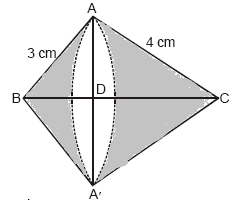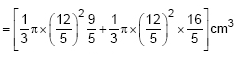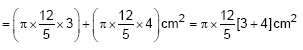Newbie

# A right triangle whose sides are 3 cm and 4 cm (other than hypotenuse) is made to revolve about its hypotenuse. Find the volume and surface area of the double cone so formed. (Choose value of π as found appropriate) Q.2

• 0

Find the solution of chapter surface areas and volumes of ncert class 10th ,give me the best solution of the exercise 13.5 question number 2 . Also find the easiest and best way to solve this tricky question .A right triangle whose sides are 3 cm and 4 cm (other than hypotenuse) is made to revolve about its hypotenuse. Find the volume and surface area of the double cone so formed. (Choose value of π as found appropriate)

Share

1. Draw the diagram as follows:Let us consider the ABA

Here,

AS = 3 cm, AC = 4 cm

So, Hypotenuse BC = 5 cm

We have got 2 cones on the same base AA’ where the radius = DA or DA’

By putting the value of CA, AB and CB we get,

We also know,

DB/AB = AB/CB

So, DB = 9/5 cm

As, CD = BC-DB,

CD = 16/5 cm

Now, volume of double cone will beSolving this we get,

V = 30.14 cm3

The surface area of the double cone will be= 52.75 cm2

• 0